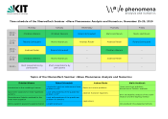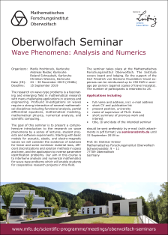Supplementary files

You can access the supplementary files with the appropriate credentials given to you during the seminar.

SchedulePoster# Oberwolfach Seminar »Wave Phenomena: Analysis and Numerics«

November 24–30, 2019

## Program

The research on wave-type problems is a fascinating and emerging field in mathematical research with many challenging applications in sciences and engineering. Profound investigations on waves require a strong interaction of several mathematical disciplines including functional analysis, partial differential equations, mathematical modeling, mathematical physics, numerical analysis, and scientific computing.

The goal of this seminar is to present a comprehensive introduction to the research on wave phenomena by a series of lectures, student projects and software experiments. Starting with basic models for acoustic, elastic, and electro-magnetic waves we will consider the existence of solutions for linear and some nonlinear material laws, efficient discretizations and solution methods in space and time, and the application to inverse parameter identification problems. Our aim in this course is to intertwine analysis and numerical mathematics for wave-type problems which will enable students for cooperative research projects in this field.

outdooractive

## Background material

In the following we list some references as background material for the lectures.

### Marlis Hochbruck

1. M. Hochbruck, J. Köhler: Error analysis of discontinuous Galerkin discretizations of a class of linear wave-type problems, Preprint, 2019.
2. M. Hochbruck, A. Sturm: Upwind discontinuous Galerkin space discretization and locally implicit time integration for linear Maxwell's equations, Math. Comp., vol. 88, no. 317, pp. 1121–1153, 2019.
3. M. Hochbruck, J. Köhler: On the efficiency of the Peaceman-Rachford ADI-dG method for wave-type methods, In: F. A. Radu, K. Kumar, I. Berre, J. M. Nordbotten, I. S. Pop (Eds.), Numerical Mathematics and Advanced Applications ENUMATH 2017. Lecture Notes in Computational Science and Engineering, vol. 126. Springer International Publishing. Pp. 135-144, 2019.
4. J. Köhler: The Peaceman–Rachford ADI-dG method for linear wave-type problems, PhD thesis, 2018.
5. A. Sturm: Locally implicit time integration for linear Maxwell's equations, PhD thesis, 2017.
6. M. Hochbruck, A. Sturm: Error analysis of a second-order locally implicit method for linear Maxwell's equations, SIAM J. Numer. Anal., vol. 54, no. 5, pp. 3167–3191, 2016.
7. T. Pažur: Error analysis of implicit and exponential time integration of linear Maxwell's equations, PhD thesis, 2013.

### Andreas Rieder

1. A. Kirsch, A. Rieder: Inverse problems for abstract evolution equations with applications in electrodynamics and elasticity, Inverse Problems 32 085001, 2016.
2. A. Kirsch, A. Rieder: Inverse problems for abstract evolution equations II: higher order differentiability for viscoelasticity, Preprint, to appear in SIAM J. Appl. Math., 2019
3. C. Grathwohl, P. Kunstmann, E. T. Quinto, A. Rieder: Microlocal analysis of imaging operators for effective common offset seismic reconstruction, Inverse Problems 34 114001, 2018.
4. C. Grathwohl, P. Kunstmann, E. T. Quinto, A. Rieder: Approximate inverse for the common offset acquisition geometry in 2D seismic imaging, Inverse Problems 34 014002, 2018.
5. U. Zeltmann: The Viscoelastic Seismic Model: Existence, Uniqueness and Differentiability with Respect to Parameters, PhD thesis, Karlsruhe Institute of Technology, 2019.

### Roland Schnaubelt

1. P. D'Ancona, S. Nicaise, R. Schnaubelt: Blow-up for nonlinear Maxwell equations, Electron. J. Differ. Eq., vol. 2018, no. 73, pp. 1-9, 2018.
2. I. Lasiecka, M. Pokojovy, R. Schnaubelt: Exponential decay of quasilinear Maxwell equations with interior conductivity, online first, to appear in NoDEA Nonlinear Differential Equations Appl..
3. M. Pokojovy, R. Schnaubelt: Boundary stabilization of quasilinear Maxwell equations, J. Differ. Equ., vol. 268, issue 2, pp. 784-812, online first, 2020.
4. R. Schnaubelt, M. Spitz: Local wellposedness of quasilinear Maxwell equations with conservative interface conditions, Preprint, 2018.
5. R. Schnaubelt, M. Spitz: Local wellposedness of quasilinear Maxwell equations with absorbing boundary conditions, Preprint, 2018.
6. M. Spitz: Local wellposedness of nonlinear Maxwell equations with perfectly conducting boundary conditions, J. Differ. Eq., vol. 266, issue 8, pp. 5012–5063, 2019.
7. M. Spitz: Regularity theory for nonautonomous Maxwell equations with perfectly conducting boundary conditions, Preprint, 2018.
8. M. Spitz: Local Wellposedness of Nonlinear Maxwell Equations, PhD thesis, Karlsruhe Institute of Technology, 2017.

### Christian Wieners

1. You will find everything here http://www.math.kit.edu/ianm3/page/mfoseminar/en.